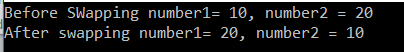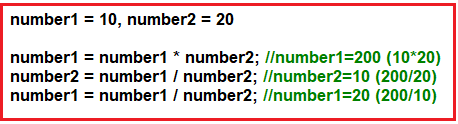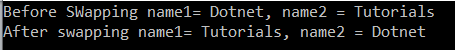# Swapping Program in C#

## Swapping Program in C# with Examples

In this article, I am going to discuss the Swapping Program in C# with and without using third variables. In many C#.NET interviews, the interviewers asked this question to write the logic to swap two numbers. As part of this article, we are going to discuss the following pointers.

1. How to swap two numbers using third variable in C#?
2. How to swap two numbers without using third variable in C#?
3. Examples using both numbers and strings.

First, we will discuss how to swap two numbers using the third variable. Then we will discuss how to swap two integers and two strings without using the third variable in C#.

##### Swapping two numbers using a third variable in C#:

In the following program, in order to swap two numbers, we use a third variable, firstly we assign the first variable to the temporary variable then assign the second variable to the first variable and finally assigns the value which is in the third variable (which holds the first number) to the second variable.

```using System;
namespace LogicalPrograms
{
public class Program
{
public static void Main()
{
int number1 = 10, number2 = 20, temp = 0;
Console.WriteLine(\$"Before SWapping number1= {number1}, number2 = {number2}");
temp = number1; //temp=10
number1 = number2; //number1=20
number2 = temp; //number2=10
Console.WriteLine(\$"After swapping number1= {number1}, number2 = {number2}");
}
}
}
```

Output:##### Swap two integers without using the third variable in C#

There are two ways you can use to swap two numbers without using the third variable:

1. By using * and /
2. By using + and –
##### Program using * and /The following program uses * and / to swaps two integer numbers without using the third variable in C#.

```using System;
namespace LogicalPrograms
{
public class Program
{
public static void Main()
{
int number1 = 10, number2 = 20;
Console.WriteLine(\$"Before SWapping number1= {number1}, number2 = {number2}");
number1 = number1 * number2; //number1=200 (10*20)
number2 = number1 / number2; //number2=10 (200/20)
number1 = number1 / number2; //number1=20 (200/10)
Console.WriteLine(\$"After swapping number1= {number1}, number2 = {number2}");
}
}
}
```

Output:##### Program using + and –

The following program uses + and – operator to swaps two integer values without using the third variable in C#.

```using System;
namespace LogicalPrograms
{
public class Program
{
public static void Main()
{
int number1 = 10, number2 = 20;
Console.WriteLine(\$"Before SWapping number1= {number1}, number2 = {number2}");
number1 = number1 + number2; //number1=30 (10+20)
number2 = number1 - number2; //number2=10 (30-20)
number1 = number1 - number2; //number1=20 (30-10)
Console.WriteLine(\$"After swapping number1= {number1}, number2 = {number2}");
}
}
}
```

It will give you the same output as the previous program.

##### Swap two Strings without using the third variable in C#.

Algorithm: To swap two strings in C#

Step1: First Append the second string with the first string and store in the first string:

String1 = String1 + String2

Step2: In step2, call the Substring Method (int startIndex, int length) by passing the startindex as 0 and length as String1.Length – String2.Length:

String2 = Substring(0, String1.Length – String2.Length);

Step3: In step3, Call the Substring Method (int startIndex) by passing the startindex as String2.Length.

String1 = Substring(String2.Length);

The following program swaps two strings without using the third variable in C#.

```using System;
namespace LogicalPrograms
{
public class Program
{
public static void Main()
{
string name1 = "Dotnet", name2 = "Tutorials";
Console.WriteLine(\$"Before SWapping name1= {name1}, name2 = {name2}");

// Step1: append 2nd string with the 1st string
name1 = name1 + name2;

//Step2: store intial string name1 in string name2
name2 = name1.Substring(0, name1.Length - name2.Length);

//Step3:  store initial string name2 in string name1
name1 = name1.Substring(name2.Length);

Console.WriteLine(\$"After swapping name1= {name1}, name2 = {name2}");
}
}
}
```

Output:As you can see, here we are using the Substring() built-in method. Let us understand this method in detail.

##### Understanding String.Substring Method in C#:

The Substring() method in C# comes in two forms. They are as follows:

String.Substring Method (startIndex): This Substring() method is used to extract a substring from the current instance of the string. The parameter “startIndex” will specify the starting position of substring and then the substring will continue to the end of the string.

String.Substring Method (int startIndex, int length): This Substring() method is used to retrieve a substring that begins from the specified position described by parameter “startIndex” and has a specified length. If the startIndex is equal to the length of string and parameter length is zero, then it will return nothing as a substring.

I hope you understood how to swap two values with and without using third variable in C#. In the next article, I am going to discuss the Fibonacci Series program in some different ways.

### 1 thought on “Swapping Program in C#”

1.We could solve this proplem like this:
(name1, name2) = (name2, name1)